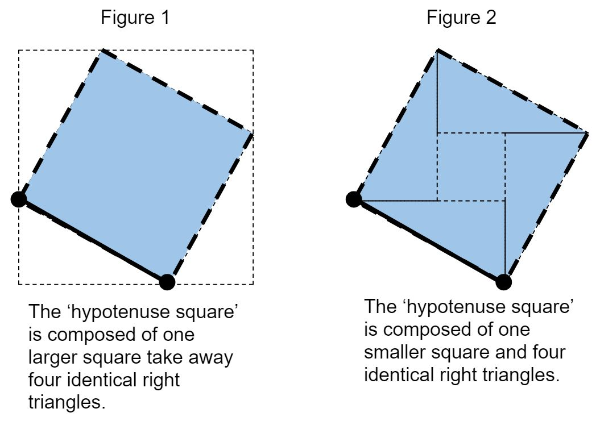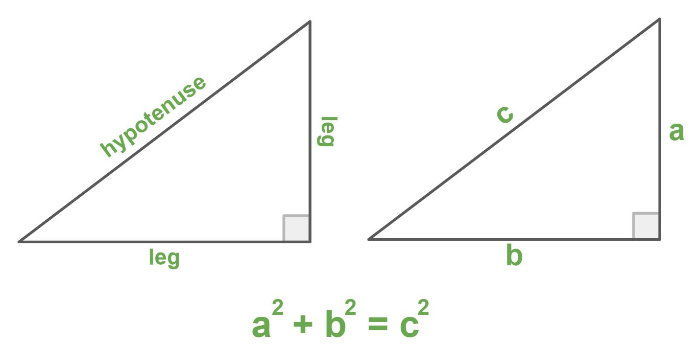# Discover Pythagorean Theorem

## Learning Goal

Students will explore relationships between squares and right triangles, and learn the Pythagorean Theorem.

## 'Hypotenuse Squares'So far, we know that we can find the distance between two points or the hypotenuse of a right triangle by: Finding a composition of shapes to make the 'hypotenuse square'Finding the area of the squareFinding the square root of the area

## Question 1: Use the Visual to...

Choose only ONE set of points, and use the 'hypotenuse square' to find the distance between them.

1. (-5, -2) and (3, 2)
2. (-3, -3) and (0, -9)
Hint: Review the 'Hypotenuse Squares' figures and explanation above.

## What's next?

Finding the distance between two points (or the length of the hypotenuse) is possible by finding the square root area of the 'hypotenuse square'. Now, we will look for a simpler way to find the area of this square.

## Question 2: Use the Visual to...

List the 3 square areas for a right triangle with each set of side lengths.

1. 3, 4, and 5 units
2. 5, 12, and 13 units
3. 6, 8, and 10 units
What patterns do you see within each list of areas? Bonus: Use the words leg and hypotenuse.

## Question 3: Use the Visual to...

Describe what is happening in your own words.

## What's next?

Finding the distance between two points (or the length of the hypotenuse) becomes more straightforward if we use the relationship between the squares of the sides of a right triangle. This relationship is called The Pythagorean Theorem and is often represented by this equation: , where and are the lengths of the legs (shorter sides) and is the length hypotenuse (longer side).

## The Pythagorean TheoremFor a right triangle, the squares of the two legs (a & b) add up to the square of the hypotenuse (c).

## Question 4: Use the Visual to...

Find the hypotenuse 'c' for each set of leg lengths ('a' & 'b').

1. a = 1, b = 7, c = ?
2. a = 9, b = 4, c = ?
Hint: Use the Pythagorean Theorem, .